# Scale Factors on the GMAT: Percent Increases and Decreases

First, try these practice questions:

1) Every length in a 45°-45°-90° triangle increases by 20%.  By what percent does the area of the triangle increase?

(A) 20%
(B) 25%
(C) 40%
(D) 44%
(E) 80%

2) At a certain pizza parlor, the diameter of a large pizza is 40% larger than the diameter of a small pizza.  What is the percent increase in total amount of pizza, from a small to a large?

(A) 20%
(B) 40%
(C) 64%
(D) 80%
(E) 96%

3) For a certain cylinder, the diameter equals the height.  If every length in this cylinder is decreased by 60%, then to the nearest integer, by what percent does the volume decrease?

(A) 22%
(B) 40%
(C) 60%
(D) 84%
(E) 94%

Do these questions stymie or confuse you?  Then you have found exactly the right post to read!

## Scale factor

In Geometry, we often “scale up” and “scale down” shapes — that is to say, we make bigger or smaller copies that have the same “shape”, but in a larger or smaller version.  This process is called dilation, but that’s a word you don’t need to know.  When we want to talk about how much bigger or how much smaller the new shape is, it’s convenient to use the idea of a scale factorThe scale factor is the factor by which every length in the shape has been multiplied.  If the shape has been scaled “up”, so that the result is larger, then the scale factor is greater than 1.  Conversely, if the shape has been scaled “down”, so that the result is smaller, then the scale factor is less than 1.  I will use the letter k for scale factor.

For example, if k = 3, that means every length in the shape has tripled; and if k = 1/5, that means every length in the shape has been divided by 5 —– of course, that would be an 80% decrease.

## Scale factor & percent change

If the appearance of the percent decrease at the end of the last section threw you for a loop, it probably means you need to catch up a little on how percents work.  See this blog.  That blog explains how percent increase and percent decrease can be expressed as a multipliers — that’s one of the most powerful mathematical tricks you can know.  In particular, when a geometric shape scales up or down by a certain percent, the percentage change multiplier is the scale factor!   That’s BIG IDEA #1 of this post.

## Change in area

Once you understand this next idea, it is one of the most blessedly easy rules in all of mathematics.  If every length is multiplied by scale factor k, then the area is multiplied by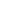.  That is BIG IDEA #2 of this post.

For example, if I increase each length by 50% — this means, I multiply by 1.5, which in turn means k = 1.5.  From this we can calculate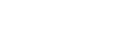— that means area goes up to 225 percent, which is an increase of 125% above the original 100%.  Thus, area would increase by 125%.

## Change in volume

Another one of the easiest mathematical laws imaginable.  If every length is multiplied by scale factor k, then the volume is multiplied by.  That is BIG IDEA #3 of this post.

For example, if each side of a box is tripled (i.e. k = 3), then total volume increases by a factor of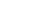.  If each side of a box is multiplied by 5, k = 5, then the total volume is multiplied by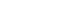.

## Summary

At this point, armed with these three “big ideas”, you may want to go back to the practice problems above and give them a second try, before reading the explanations below.

## Practice problem solutions

1) The irony of this question is, of course, the specifics of the shape don’t matter at all.  Yes, in general it is a good idea to be familiar with the 45°-45°-90° triangle —- if you’re not, see this post —– but none of that matters here.

We have a 20% increase, so that’s a multiplier of 1.2, which means k = 1.2.  The multiplier for area would be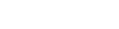, which is the multiplier for a 44% increase.  That’s the percent increase in area.  Answer = D

2) A real world problem —- yum! —- but again, the specifics of the shape don’t matter.  Diameter increases 40%, so k = 1.4 in this case.  “Amount of pizza” just means the area of the circle, so, which is the multiplier for a 96% increase.  That’s the percent increase in area.  Answer = E

3) Again, the exact dimensions of the cylinder do not matter, as long as every length increases by the same percent or same ratio.  We now have a percent decrease, which means we subtract to construct our multiplier.  A 60% decrease means we have a multiplier of 1 – 0.60 = 0.4, and so k = 0.4 as well.  For volume we need to cube the scale factor: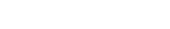approximately.  That would be the multiplier for a 94% decrease, because 1 – 0.94 = 0.06; therefore, the volume decreases by 94%.  Answer = E.

## Author

•Mike served as a GMAT Expert at Magoosh, helping create hundreds of lesson videos and practice questions to help guide GMAT students to success. He was also featured as "member of the month" for over two years at GMAT Club. Mike holds an A.B. in Physics (graduating magna cum laude) and an M.T.S. in Religions of the World, both from Harvard. Beyond standardized testing, Mike has over 20 years of both private and public high school teaching experience specializing in math and physics. In his free time, Mike likes smashing foosballs into orbit, and despite having no obvious cranial deficiency, he insists on rooting for the NY Mets. Learn more about the GMAT through Mike's Youtube video explanations and resources like What is a Good GMAT Score? and the GMAT Diagnostic Test.